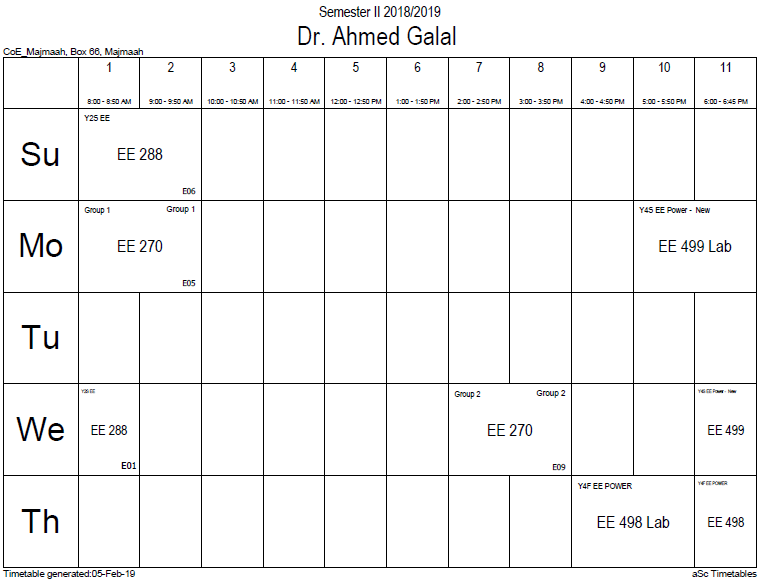# Signal-to-noise ratio (often abbreviated SNR or S/N) is a measure used in science and engineering that compares the level of a desired signal to the level of background noise. It is defined as the ratio of signal power to the noise power. A ratio higher than 1:1 indicates more signal than noise. While SNR is commonly quoted for electrical signals, it can be applied to any form of signal (such as isotope levels in an ice core or biochemical signaling between cells). The signal-to-noise ratio, the bandwidth, and the channel capacity of a communication channel are connected by the Shannon–Hartley theorem. Signal-to-noise ratio is sometimes used informally to refer to the ratio of useful information to false or irrelevant data in a conversation or exchange. For example, in online discussion forums and other online communities, off-topic posts and spam are regarded as "noise" that interferes with the "signal" of appropriate discussion.

## Definition

Signal-to-noise ratio is defined as the power ratio between a signal (meaningful information) and the background noise (unwanted signal):$mathrm{SNR} = frac{P_mathrm{signal}}{P_mathrm{noise}},$

where P is average power. Both signal and noise power must be measured at the same or equivalent points in a system, and within the same system bandwidth. If the signal and the noise are measured across the same impedance, then the SNR can be obtained by calculating the square of the amplitude ratio:$mathrm{SNR} = frac{P_mathrm{signal}}{P_mathrm{noise}} = left ( frac{A_mathrm{signal}}{A_mathrm{noise} } ight )^2,$

where A is root mean square (RMS) amplitude (for example, RMS voltage). Because many signals have a very wide dynamic range, SNRs are often expressed using the logarithmic decibel scale. In decibels, the SNR is defined as$mathrm{SNR_{dB}} = 10 log_{10} left ( frac{P_mathrm{signal}}{P_mathrm{noise}} ight ) = {P_mathrm{signal,dB} - P_mathrm{noise,dB}},$

which may equivalently be written using amplitude ratios as$mathrm{SNR_{dB}} = 10 log_{10} left ( frac{A_mathrm{signal}}{A_mathrm{noise}} ight )^2 = 20 log_{10} left ( frac{A_mathrm{signal}}{A_mathrm{noise}} ight ).$

The concepts of signal-to-noise ratio and dynamic range are closely related. Dynamic range measures the ratio between the strongest un-distorted signal on a channel and the minimum discernable signal, which for most purposes is the noise level. SNR measures the ratio between an arbitrary signal level (not necessarily the most powerful signal possible) and noise. Measuring signal-to-noise ratios requires the selection of a representative or reference signal. In audio engineering, the reference signal is usually a sine wave at a standardized nominal or alignment level, such as 1 kHz at +4 dBu (1.228 VRMS).

SNR is usually taken to indicate an average signal-to-noise ratio, as it is possible that (near) instantaneous signal-to-noise ratios will be considerably different. The concept can be understood as normalizing the noise level to 1 (0 dB) and measuring how far the signal 'stands out'.

### Office HoursNo office hours### My Timetable### Contactsemail: [email protected]

Phone: 2570

### Welcome

Welcome To Faculty of Engineering### IEEEhttp://www.ieee.org/

/

### Bookmarkshttp://www.utk.edu/research/

http://science.doe.gov/grants/index.asp

http://www1.eere.energy.gov/vehiclesandfuels/

http://www.eere.energy.gov/

### Upcoming Conferences### Engineering quotes### Travel Web Siteshttp://www.hotels.com/

http://www.orbitz.com/

http://www.hotwire.com/us/index.jsp

http://www.kayak.com/

### Blackboardستقام اختبارات الميدتيرم يوم الثلاثاء 26-6-1440

حسب الجدول المعلن بلوحات الاعلان

### Summer trainingThe registration for summer training will start from 5th week of second semesterClass registration week 1

### برنامج التجسير### إحصائية الموقع

عدد الصفحات: 2879

البحوث والمحاضرات: 1280

الزيارات: 101044